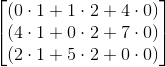# Linear Algebra : Matrix-Matrix Product

## Example Questions

← Previous 1 3 4 5 6 7 8 9 10 11

### Example Question #681 : Linear Algebra

Compute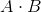where,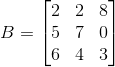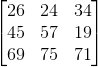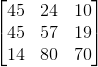Not Possibe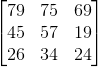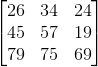Explanation:

Since the number of columns in the first matrix equals the number of rows in the second matrix, we know that these matrices can be multiplied together.  To determine the dimensions of the product matrix, we take the number of rows in the first matrix and the number of columns in the second matrix.  For this example, our product matrix will have dimensions of (3x3). The product matrix equals,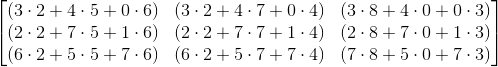### Example Question #1 : Matrix Matrix Product

Computewhere,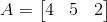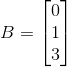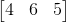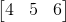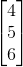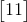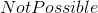Explanation:

Since the number of columns in the first matrix equals the number of rows in the second matrix, we know that these matrices can be multiplied together.  To determine the dimensions of the product matrix, we take the number of rows in the first matrix and the number of columns in the second matrix.  For this example, our product matrix will have dimensions of (1x1).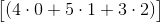### Example Question #3 : Matrix Matrix Product

Computewhere,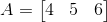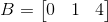Not PossibleNot Possible

Explanation:

In order to be able to multiply matrices, the number of columns of the 1st matrix must equal the number of rows in the second matrix.  Here, the first matrix has dimensions of (1x3). This means it has one row and three columns.  The second matrix has dimensions of (1x3), also one row and three columns.  Since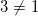, we cannot multiply these two matrices together

### Example Question #4 : Matrix Matrix Product

Compute, where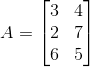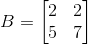Not PossibleExplanation:

Since the number of columns in the first matrix equals the number of rows in the second matrix, we know that these matrices can be multiplied together.  To determine the dimensions of the product matrix, we take the number of rows in the first matrix and the number of columns in the second matrix.  For this example, our product matrix will have dimensions of (3x2). The product matrix equals,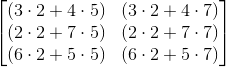### Example Question #5 : Matrix Matrix Product

Computewhere,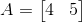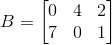Not PossibleExplanation:

Since the number of columns in the first matrix equals the number of rows in the second matrix, we know that these matrices can be multiplied together.  To determine the dimensions of the product matrix, we take the number of rows in the first matrix and the number of columns in the second matrix.  For this example, our product matrix will have dimensions of (1x3). The product matrix equals,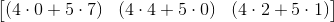### Example Question #6 : Matrix Matrix Product

Computewhere,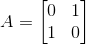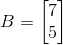Not PossibleExplanation:

Since the number of columns in the first matrix equals the number of rows in the second matrix, we know that these matrices can be multiplied together.  To determine the dimensions of the product matrix, we take the number of rows in the first matrix and the number of columns in the second matrix.  For this example, our product matrix will have dimensions of (2x1). The product matrix equals,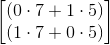### Example Question #7 : Matrix Matrix Product

Computewhere,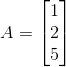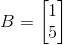Not PossibleNot Possible

Explanation:

Since the number of columns in the first matrix does not equal the number of rows in the second matrix, you cannot multiply these two matrices.

### Example Question #8 : Matrix Matrix Product

Computewhere,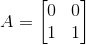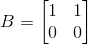Not PossibleExplanation:

Since the number of columns in the first matrix equals the number of rows in the second matrix, we know that these matrices can be multiplied together.  To determine the dimensions of the product matrix, we take the number of rows in the first matrix and the number of columns in the second matrix.  For this example, our product matrix will have dimensions of (2x2). The product matrix equals,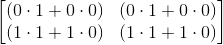### Example Question #1 : Matrix Matrix Product

Computewhere,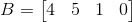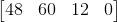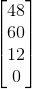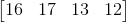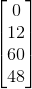Explanation:

Since the number of columns in the first matrix equals the number of rows in the second matrix, we know that these matrices can be multiplied together.  To determine the dimensions of the product matrix, we take the number of rows in the first matrix and the number of columns in the second matrix.  For this example, our product matrix will have dimensions of (1x4). The product matrix equals,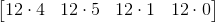### Example Question #10 : Matrix Matrix Product

Computewhere,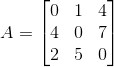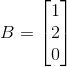Not PossibleSince the number of columns in the first matrix equals the number of rows in the second matrix, we know that these matrices can be multiplied together.  To determine the dimensions of the product matrix, we take the number of rows in the first matrix and the number of columns in the second matrix.  For this example, our product matrix will have dimensions of (3x1). The product matrix equals,